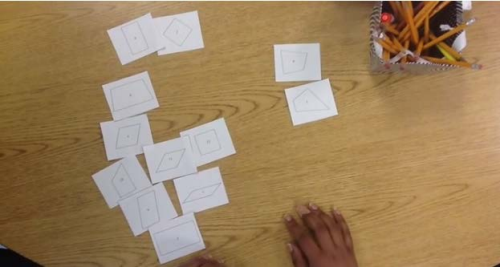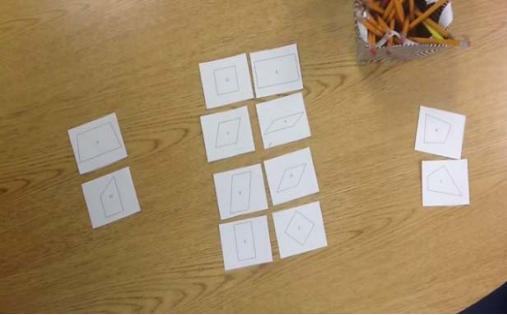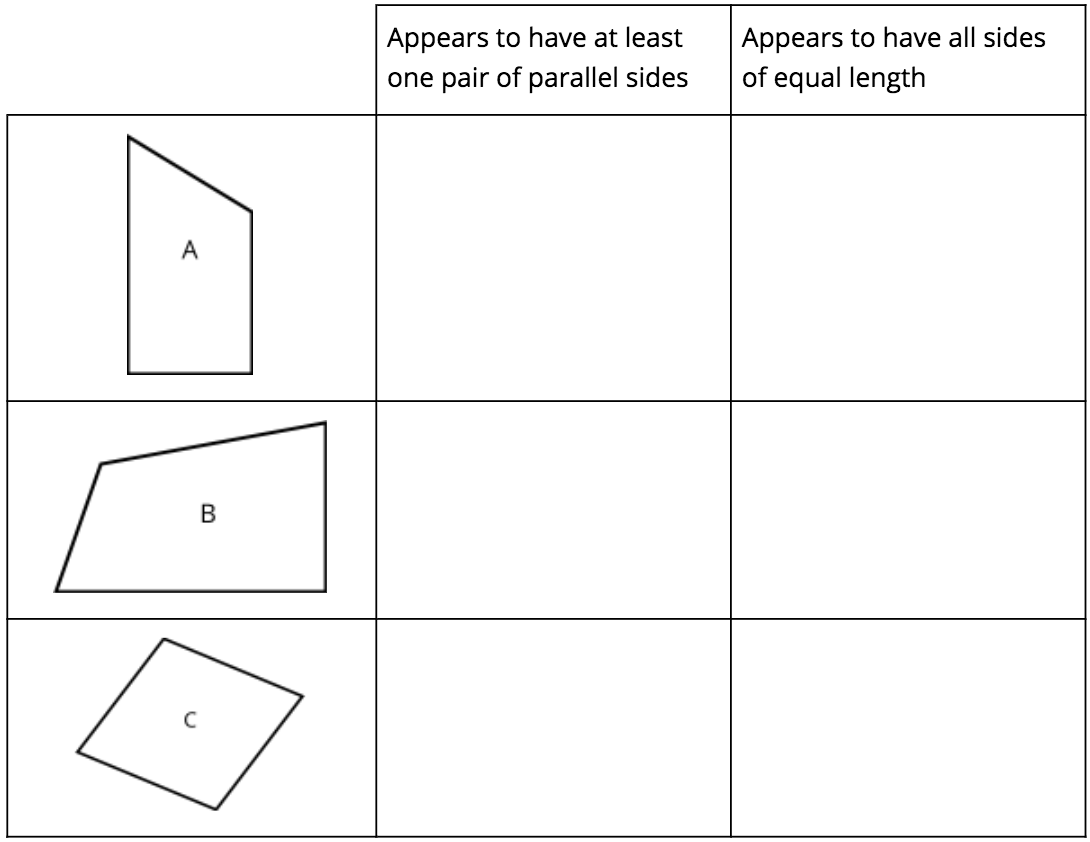# Shapes and Angles

## Objective

Analyze and classify quadrilaterals based on parallel and perpendicular lines and the presence or absence of angles of a specified size.

## Common Core Standards

### Core Standards

?

• 4.G.A.1 — Draw points, lines, line segments, rays, angles (right, acute, obtuse), and perpendicular and parallel lines. Identify these in two-dimensional figures.

• 4.G.A.2 — Classify two-dimensional figures based on the presence or absence of parallel or perpendicular lines, or the presence or absence of angles of a specified size. Recognize right triangles as a category, and identify right triangles.

?

• 3.G.A.1

## Criteria for Success

?

1. Make sense of a three-act task and persevere in solving it (MP.1).
2. Classify/sort quadrilaterals according to their attributes like side length, angle measure, and presence of parallel/perpendicular sides (MP.2, MP.5).
3. Understand that a quadrilateral is a closed shape with four straight sides.
4. Understand that a trapezoid is a quadrilateral with at least one pair of parallel sides. (See Notes/Tips for Teachers section.)
5. Understand that a parallelogram is a quadrilateral with exactly two pairs of parallel sides.
6. Understand that a rectangle is a parallelogram with perpendicular sides.
7. Understand that a rhombus is a parallelogram with four sides of equal length.
8. Understand that a square is a parallelogram with perpendicular sides of equal length.
9. Understand that shapes can be classified into more than one category.

## Tips for Teachers

?

• Students have learned all of the vocabulary used in today’s lesson in prior grade levels. Thus, today’s lesson serves to refine the definitions of that vocabulary and engage with this content more deeply, given students’ new understanding of angles from work throughout this unit.
• “In the U.S., that the term ‘trapezoid’ may have two different meanings. In their study The Classification of Quadrilaterals (Information Age Publishing, 2008), Usiskin et al. call these the exclusive and inclusive definitions:
• T(E): a trapezoid is a quadrilateral with exactly one pair of parallel sides
• T(I): a trapezoid is a quadrilateral with at least one pair of parallel sides.

These different meanings result in different classifications at the analytic level. According to T(E), a parallelogram is not a trapezoid; according to T(I), a parallelogram is a trapezoid. Both definitions are legitimate. However, Usiskin et al. conclude, ‘The preponderance of advantages to the inclusive definition of trapezoid has caused all the articles we could find on the subject, and most college-bound geometry books, to favor the inclusive definition.’” (Geometry Progression, p. 3). Thus, the inclusive definition is used below and throughout the curriculum.

### Lesson Materials

• Ruler (1 per student) — This can be any tool used to verify equal lengths, e.g., the edge of a piece of paper, etc.
• 180° Protractor (1 per student)
• Template: Quadrilaterals (1 per student or small group) — This material should be cut into pieces before the lesson.

#### Fishtank Plus

• Problem Set
• Student Handout Editor
• Vocabulary Package

?

### Problem 1

Act 1: Watch the video: Investigating Quadrilaterals.

How is this person sorting the cards each time?

#### References

Investigating Quadrilaterals by Jenise Sexton is made available on 101Questions under the CC BY 3.0 license. Accessed Feb. 5, 2018, 12:05 p.m..

Modified by Fishtank Learning, Inc.

### Problem 2

Act 2: Use the following information to solve.

 First sort:Second sort:#### References

Investigating Quadrilaterals by Jenise Sexton is made available on 101Questions under the CC BY 3.0 license. Accessed Feb. 5, 2018, 12:05 p.m..

Modified by Fishtank Learning, Inc.

### Problem 3

• The shapes were first sorted according to whether they had at least one set of parallel sides or not.
• The shapes were then sorted according to whether they had two pairs of parallel sides or not.
 First sort:Second sort:#### References

Investigating Quadrilaterals by Jenise Sexton is made available on 101Questions under the CC BY 3.0 license. Accessed Feb. 5, 2018, 12:05 p.m..

Modified by Fishtank Learning, Inc.

### Problem 4

Act 4 (the sequel): Sort the quadrilaterals from Template: Quadrilaterals in a different way from how they were sorted in the three-act task above.

#### References

Investigating Quadrilaterals by Jenise Sexton is made available on 101Questions under the CC BY 3.0 license. Accessed Feb. 5, 2018, 12:05 p.m..

## Problem Set & Homework

### Discussion of Problem Set

• How is it possible for the shape in #1 to be a trapezoid, but no side to be parallel to side $r$?
• What was the most specific word you used for each part of #2? What other terms would work?
• For #3, what makes a square different from a rectangle? Why is it important to define a square as a rectangle with four equal-length sides and not as a quadrilateral with four equal-length sides?
• What is the most specific term you could use to describe the shape you chose in #6? Are there other answer choices that could be described with the same word?
• What are some attributes that every square has in common? How is a square a special case of a rectangle, a parallelogram, and a trapezoid?
• If your teacher asked you to draw a trapezoid and you drew a parallelogram, explain to your teacher why a parallelogram is also a trapezoid.

?

### Problem 1

For each figure in the table below, determine whether each statement describes the figure. If so, place an X in the box. You may mark more than one box for each figure.### Problem 2

Based on the properties identified in the table above and any other properties you can identify, name each shape, being as specific as possible. Write the name of each shape below.

Shape A: ______________

Shape B: ______________

Shape C: ______________